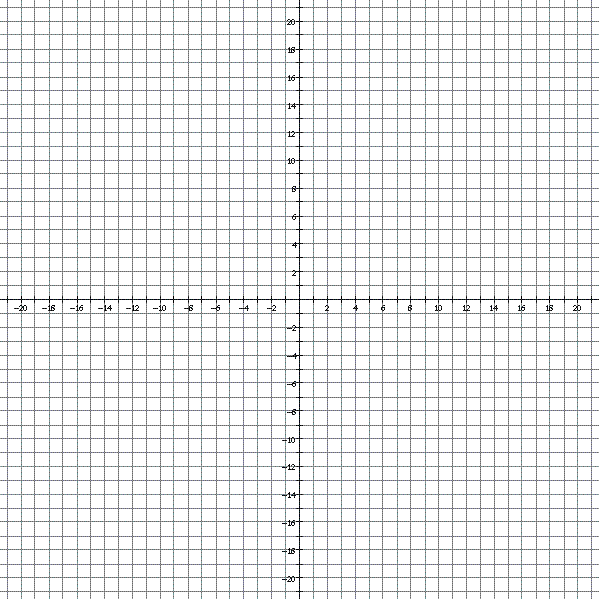Name:_____________________________________Date:__________________Per:______Graph all of the following linear equations (linear functions) on the coordinate
grid above using the slope and y-intercept to make the graphs.

 1. y = -3x - 12 6. y = 2x - 20 2. y = -3x - 6 7. y = 2x - 16 3. y = -3x 8. y = 2x - 12 4. y = -3x + 6 9. y = 2x - 8 5. y = -3x + 12 10. y = 2x - 4